Vibroengineering PROCEDIA

Published: 26 November 2020

# Control of the air suspension system of semi-trailer trucks to enhance the road friendliness

Huaxiang Zhou1
Xiaoyan Wu2
Peiling Wang3
1, 2, 3School of Mechanical and Electrical Engineering, Hubei Polytechnic University, Huangshi, 435003, China
1, 2, 3Hubei Key Laboratory of Intelligent Conveying Technology and Device (Preparatory), Hubei Polytechnic University, Huangshi 435003, China
Corresponding Author:
Xiaoyan Wu
Views 257

#### Abstract

An air suspension system of semi-trailer trucks controlled by semi-active fuzzy logic control is proposed and researched to reduce the dynamic load coefficient (DLC) of the wheel axles. A dynamical model described by a nonlinear system with 7-DOF of the vehicle and a Simulink program developed in Matlab software are applied to simulate the DLC values under different operation conditions. The fuzzy control is then applied to control damping coefficients of the air suspension system. The obtained results show that semi-active air suspension of semi-trailer truck is overall superior to passive air suspension. The DLC values are also significantly decreased in all cases of the road surface roughness, over a range of vehicle velocity and the different loaded conditions. Particularly, under vehicle fully loaded condition, the DLC value of the tractor driver is greatly reduced by 27.4 % at speed of 27.5 m/s on the ISO level B road.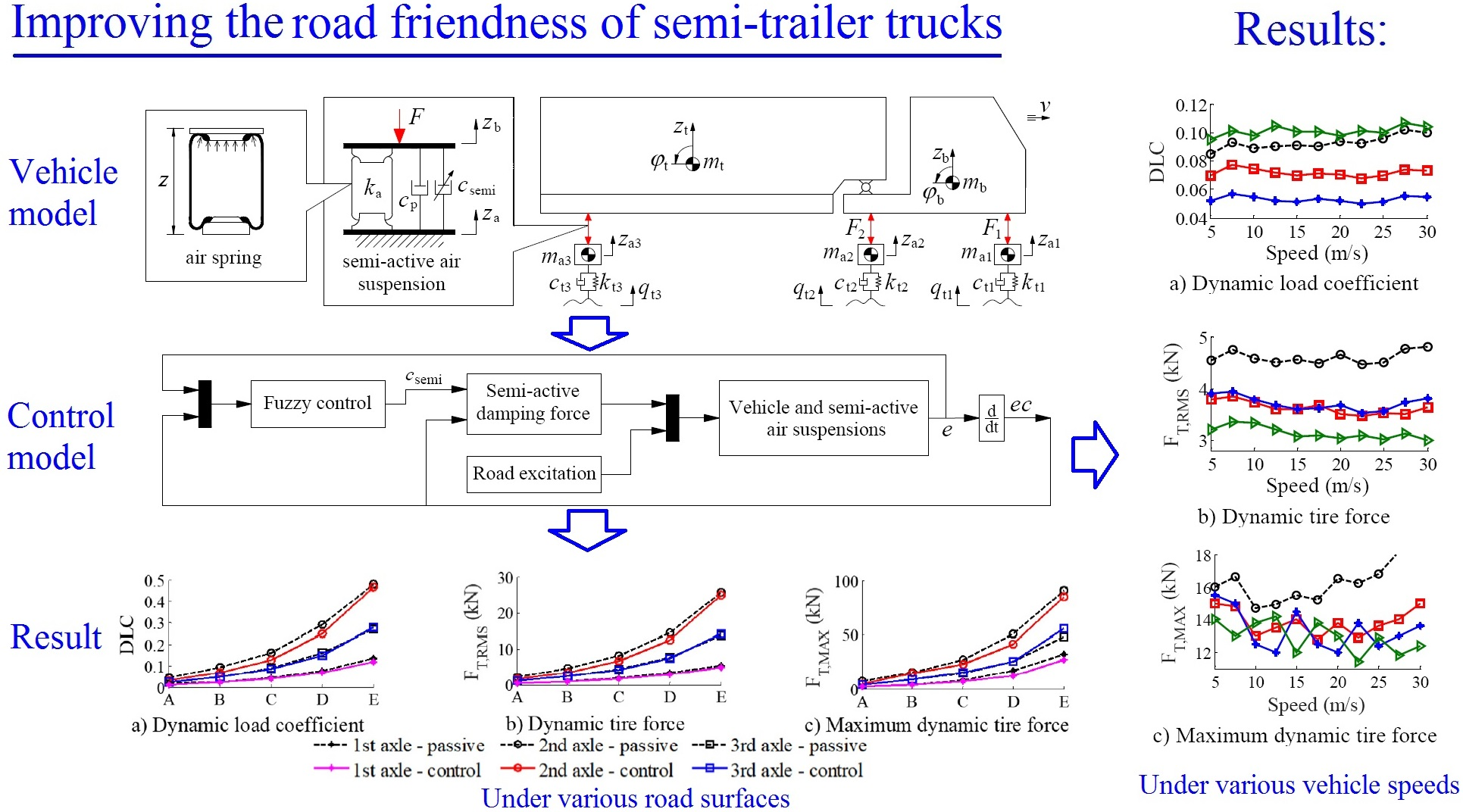#### Highlights

• The fuzzy control is then applied to control damping coefficients of air suspension system of heavy trucks to improve the road friendness.
• Semi-active air suspension of semi-trailer truck is overall superior to passive air suspension.
• The DLC values are also significantly decreased in all case of the road surface roughness, over a range of vehicle velocity and different loaded conditions.
• Under vehicle fully loaded condition, the DLC value of the tractor driver is greatly reduced by 27.4% at speed of 27.5 m/s on the ISO level B road.

## 1. Introduction

The ride comfort and road friendliness are two important indexes to evaluate the working performance of the suspension system of the semi-trailer trucks. To improve the ride comfort and road friendliness, the vehicle’s suspension system ought to be able to isolate the sprung mass from road-induced disturbances and reduce the dynamic load coefficient (DLC) of tire forces from vehicle axles within limit of a set working space under various operating conditions. In former times, passive suspensions were mostly used in the semi-trailer trucks. They are now widely studied and improved by using air suspensions, active and semi-active suspensions due to their capabilities of consuming less power, low cost, and providing better ride quality. Many researchers showed that the application of the active or semi-active suspension for vehicles could improve the ride quality and reduce dynamic tire forces [1-3]. Some control methods of the fuzzy control [4, 5], FLC-skyhook damper, FLC-PID control [6, 7], FLC-Hinf control , Optimal fuzzy control [9, 10], or Skyhook-Neuro fuzzy control  were used to adjust the damping coefficient of active or semi-active suspension via the quarter car model. A half-heavy truck model was then applied to control the tractor driver suspensions . The control performance between the damping coefficient and stiffness of air spring of heavy trucks was researched based on the optimal fuzzy control . The main goals of the researches were to improve ride comfort. The researches also noted that the reduction of the dynamical force on wheel axles could significantly reduce road damage.

The air suspensions with air springs are preferred choice instead of conventional steel springs. This is because the former can effectively reduce the effect of the disturbance from the road input and also easily adjust the ride height on board. The performance of air suspensions of heavy trucks compared to steel suspensions was evaluated via the DLC value of the wheel axles . The results showed that the DLC value was greatly decreased by using the air suspensions. The FLC-PID control used for the semi-active air suspension of heavy vehicles was also studied. The ride comfort and tire loads were significantly improved . All the results showed that the heavy truck's semi-active suspension system not only increases the ride comfort but also reduces the road damage. However, the effect of various operating conditions on the road friendliness had not yet been considered in these studies. Thus, the performance of semi-active air suspensions of the vehicles on the road friendliness was not fully reflected either.

In this study, a dynamic model of a semi-trailer truck is established. The fuzzy control is applied to control the semi-active air suspension system. Matlab software is then used to simulate and calculate the DLC value and dynamic load of tires under different operation conditions including the road surface roughness, vehicle loading, and vehicle velocity. The aim of this study is to improve road friendliness and reduce road damage to the vehicle.

## 2. The model of semi-trailer truck with a semi-active air suspension system

### 2.1. Semi-trailer truck dynamic model

The structure of semi-trailer trucks includes the tractor driver, trailer, and three axles of the vehicle (steering, tractor driver, and trailer axles). A dynamic model of a semi-trailer truck with 7 degrees of freedom is then established as in Fig. 1, where, ${m}_{b}$ and ${m}_{t}$ are the sprung mass of the tractor driver and trailer. ${m}_{a1,2,3}$ are the unsprung mass of the steering axle, tractor driver axle, and trailer axle. ${z}_{b}$, ${z}_{t}$, and ${z}_{a1,2,3}$ are the vertical displacements of tractor driver, trailer, and vehicle axles. ${\phi }_{b}$ and ${\phi }_{t}$ are angular displacements of tractor driver and trailer. ${q}_{t1,2,3}$ is the excitation of the road surface roughness at tires.

Fig. 1A dynamic model of semi-trailer truck with semi-active air suspension system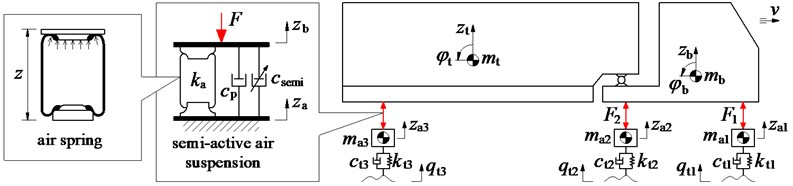Based on the vehicle dynamic model in Fig. 1 and by applying Newton’s second law, the motion equations of the vehicle can be represented in the matrix form as follows:

1
$M\stackrel{¨}{Z}+C\stackrel{˙}{Z}+KZ={F}_{q}\left(t\right),$

where $M$, $C$, and $K$ are the mass, damping, and stiffness matrices of vehicle, $Z$ is the displacement vector, and ${F}_{q}\left(t\right)$ is the force vector of the vibration excitation of the road surface.

### 2.2. Semi-active air suspension model

The air suspensions of semi-trailer trucks are used by the rolling lobe air springs and viscous dampers, and viscous dampers are controlled by semi-active fuzzy control, as modeled in the same Fig. 1.

The equation dynamic force of the air suspension system is written as follows:

2

where, ${k}_{a}$ is the stiffness coefficient of air spring. ${c}_{p}$ is the passive dumping coefficient. ${c}_{semi}$ is the semi-active damping coefficient. $z={z}_{a}-{z}_{b}$ is the relative displacement of air suspension.

Based on the calculated result of the air spring in Ref. , the ${k}_{a}$ is determined by:

3
${{k}_{a}}_{i}=\left[\left({p}_{0}+{p}_{a}\right){\left(\frac{{V}_{0}}{{V}_{0}-\alpha {z}_{i}}\right)}^{n}-{p}_{a}\right]\beta +\left[n\left({p}_{0}+{p}_{a}\right)\left(\frac{{A}_{0}+\beta {z}_{i}}{{V}_{0}-\alpha {z}_{i}}\right){\left(\frac{{V}_{0}}{{V}_{0}-\alpha {z}_{i}}\right)}^{n}\right]\alpha ,$

where, ${A}_{0}$ and ${V}_{0}$ are the initial effective area and volume, $\beta$ and $\alpha$ are the change of the effective area and volume with respect to $z$, ${p}_{0}$ and ${p}_{a}$ are the air pressure of the initial state and the standard atmospheric pressure, $n$ is a specific heat ratio.

## 3. Model of fuzzy logic control

To control semi-active air suspensions, the fuzzy control model designed for semi-active air suspensions of semi-trailer truck is shown in Fig. 2.

Fig. 2Schematics of the fuzzy logic control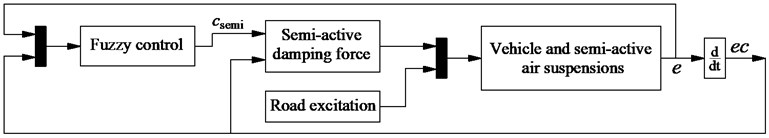Table 1Fuzzy linguistic values

 LV Description $e$ $ec$ LV ${c}_{semi}$ $pb$ Positive big 0.3 0.75 ${u}_{1}$ 3 $pm$ Positive medium 0.2 0.5 ${u}_{2}$ 6 $ps$ Positive small 0.1 0.25 ${u}_{3}$ 9 $ze$ Zero 0 0 ${u}_{4}$ 12 $ns$ Negative small –0.1 –0.25 ${u}_{5}$ 15 $nm$ Negative medium –0.2 –0.5 ${u}_{6}$ 18 $nb$ Negative big –0.3 –0.75 ${u}_{7}$ 21

Table 2Control rules

 ${c}_{semi}$ $e$ $nb$ $nm$ $ns$ $ze$ $ps$ $pm$ $pb$ $nb$ ${u}_{5}$ ${u}_{7}$ ${u}_{6}$ ${u}_{5}$ ${u}_{4}$ ${u}_{3}$ ${u}_{3}$ $nm$ ${u}_{2}$ ${u}_{5}$ ${u}_{7}$ ${u}_{5}$ ${u}_{3}$ ${u}_{3}$ ${u}_{3}$ $ns$ ${u}_{1}$ ${u}_{1}$ ${u}_{5}$ ${u}_{5}$ ${u}_{3}$ ${u}_{2}$ ${u}_{2}$ $ec$ $ze$ ${u}_{1}$ ${u}_{1}$ ${u}_{1}$ u5 ${u}_{1}$ ${u}_{1}$ ${u}_{1}$ $ps$ ${u}_{2}$ ${u}_{2}$ ${u}_{3}$ ${u}_{5}$ u5 ${u}_{1}$ ${u}_{1}$ $pm$ ${u}_{3}$ ${u}_{3}$ ${u}_{3}$ ${u}_{5}$ ${u}_{7}$ ${u}_{5}$ ${u}_{2}$ ${u}_{5}$ ${u}_{5}$ ${u}_{5}$ ${u}_{5}$ ${u}_{5}$ ${u}_{5}$ ${u}_{5}$ ${u}_{5}$

The fuzzy control consists of a fuzzification interface, a fuzzy inference system, and a defuzzification interface. First, the crisp values in fuzzification are transformed into linguistic variables (LV). The fuzzy inference system is then used by fuzzy rule in accordance with the inference rule. Finally, the linguistic variables are transformed back to crisp values through defuzzification for use by the physical plant [7, 10]. In this study, the relative displacement $e$ of $z={z}_{a}-{z}_{b}$ and its velocity $ec$ feedback signals of air suspension are considered as two input variables, while the damping coefficient ${c}_{semi}$ is the resulting output of the controller. The seven linguistic terms are defined in the Table 1. The shape of the membership function for the variables of in-output is the Triangular function and their values are between 0 and 1.

If-then rules of Fuzzy control are applied to describe according to expertise experiences and designer’s knowledge, the fuzzy rules in Table 2 are described by (1) If $e=nb$ and $ec=nb$ then ${c}_{semi}={u}_{5}$, (2) if $e=nb$ and $ec=nm$ then ${c}_{semi}={u}_{2}$, ...., and (3) if $e=pb$ and $ec=pb$ then ${c}_{semi}={u}_{5}$.

The fuzzy inference system is selected by the minimum function and centroid method of Madani . This study, Mamdani’s fuzzy inference system is used for the system model.

## 4. Vibration excitation and evaluation criteria

### 4.1. Vibration excitations

To establish the random road surface, the white noise speed spectrum represented with a realization of a random process via its frequency spectrum density (FSD) is applied to describe the random road surface. Its equation can be expressed as follows :

4
$\stackrel{˙}{q}\left(t\right)+\gamma {v}^{2}q\left(t\right)=\gamma \sqrt{S\left({n}_{0}\right)v}w\left(t\right),$

where, $\gamma$ is the spatial frequency of the road surface, $w\left(t\right)$ is the random signal of the white noise, $S\left({n}_{0}\right)$ is the road roughness power spectrum density (PSD) chosen via ISO 8068 . Based on ISO 8068, the PSD of road surface roughness of levels A, B, C, D, and E at $v=$ 20 m/s of the vehicle is chosen to simulate the excitation of the road surface roughness. The high roughness of the road surface with the simulation time of 50 s is shown in Fig. 3.

### 4.2. Evaluation criteria of road friendliness

Dynamic load coefficient (DLC) is frequently used to characterize the dynamic loading of the axles. It is defined by a ratio of the root-mean-square (RMS) of the dynamic load fluctuation over the static load . The expression of the DLC is written by $DLC={F}_{T,RMS}/{F}_{s}$. Herein, ${F}_{T,RMS}$ is the vertical RMS dynamic wheel load, ${F}_{s}$ is the average or nominal static vertical wheel load. The DLC value depends on several factors, such as road surface condition, type of suspension, vehicle velocity, load, mass, and stiffness distribution of the vehicle's structure. In general, it is in the range of 0.05 to 0.3 under normal operating conditions. It may reach to the zero when the wheels of a heavy truck are moving on a special smooth road or increase up to 0.4 when the contact between the wheels and the road surface axles is broken [9, 15]. In this study, the DLC is used to evaluate road friendliness.

## 5. Result and analysis

### 5.1. Control of air suspensions on ISO level B

As clearly discussed in the previous sections, the paper aim is to reduce the DLC values of tire forces from heavy truck axles by controlling the semi-active air suspension system on the road surface roughness. The dynamic parameters of the vehicle are used in Ref. . The fuzzy control tools in the Matlab software are then applied and designed to control the air suspensions of the vehicle under a road surface roughness of ISO level B at a speed of 20 m/s. The simulation results are shown in Fig. 4.

Fig. 3Excitation of road surface roughness according to ISO 8068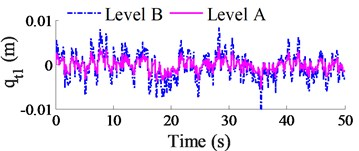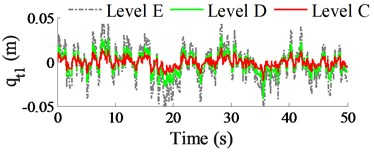Fig. 4Control results of csemi and dynamic force of tire at second axle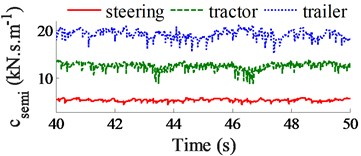a) Semi-active damping coefficients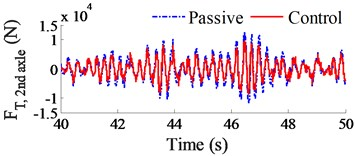b) Dynamic tire force

Fig. 4(a) is the damping coefficients of the air suspension system at the steering, tractor, and trailer axles controlled by fuzzy control, while Fig. 4(b) is the dynamic tire force on the tractor driver axle. Similar to the research results in Ref. , the dynamic tire force at 2nd axle is the largest, thus, it has a great influence on road friendliness. Observing Fig. 4(b), the dynamic tire force was significantly reduced by semi-active air suspension in comparison with its passive. The calculation results in Table 3 indicate that the DLC value at 2nd axle with the control is reduced by 22.2 % compared to the passive. Besides, the RMS and MAX of dynamic tire forces (${F}_{T,RMS}$ and ${F}_{T,MAX}$) are also significantly reduced by 23.2 % and 14.3 % in comparison with the passive. Consequently, the semi-trailer truck with semi-active air suspensions has an obvious effect on the reduction the road damage and improves the road friendliness. To fully reflect the effectiveness of semi-active air suspensions, various road surfaces are then simulated.

### 5.2. Control of air suspension under various road surfaces

Five road surfaces of ISO level A (very good) to ISO level E (very poor) are chosen to simulate and analyze the effect of road surface roughness on the effectiveness of semi-active air suspension system. The results of the DLC value, the ${F}_{T,RMS}$ and ${F}_{T,MAX}$ at each axle are shown in Fig. 5. It can see that all their values are significantly reduced in comparison with the passive. Moreover, the DLC value, the ${F}_{T,RMS}$, and ${F}_{T,MAX}$ at tractor driver axle are the maximum. Thus, the impact of tractor driver axle on road damage are the greatest. In Table 4, the DLC value of the tractor driver axle on road surface from ISO level A to ISO level D is from 0.03 to 0.25. Thus, the DLC value of vehicle is under normal operating conditions . However, the DLC value greatly increased on ISO level E road, and its value is 0.47. In this case, the wheels of the trailer driver axle spend a significant proportion of their time disconnecting the road surface.

Table 3The simulation results on ISO level B

 Parameter Passive Control Reduction DLC 0.09 0.07 22.2 % ${F}_{T,RMS}$ at2nd axle (kN) 4.62 3.55 23.2 % ${F}_{T,MAX}$ at2nd axle (kN) 15.25 13.07 14.3 %

Table 4The DLC results on the tractor axle

 Road level Value of DLC Passive Control Reduction Level A 0.04 0.03 25.0 % Level B 0.09 0.07 22.2 % Level C 0.18 0.13 27.7 % Level D 0.29 0.25 13.8 % Level E 0.52 0.47 9.6 %

Fig. 5The DLC and the dynamic tire force under the various road surfaces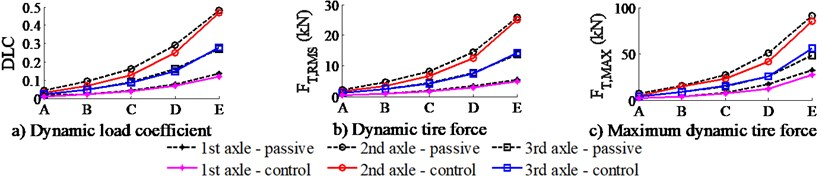Fig. 6The dynamic tire forces on the tractor driver axle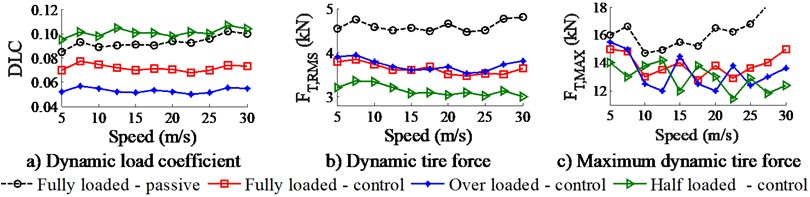### 5.3. Control of air suspension under various vehicle speeds

The simulation of semi-active air suspensions over a range of vehicle velocities under different loaded conditions (half loaded, fully loaded and over 50 % loaded) on ISO lever B road are plotted in Fig. 6. As shown in Fig. 6(a), under fully loaded-passive condition, the DLC value is increased with the increase of the vehicle speed. However, the DLC with semi-active air suspensions is significantly reduced. In Table 5, the maximum values of DLC at 7.5, 20, and 27.5 m/s are reduced by 17.9 %, 22.2 %, and 27.4 % respectively. Besides, the DLC also strongly depends on the loaded condition, the DLC is greatly increased in the case of the half loaded-control and it is also greatly reduced in the case of over 50 % loaded-control in comparison with the fully load-control of the trailer.

Both the ${F}_{T,RMS}$ and ${F}_{T,MAX}$ on the tractor driver axle in Fig. 10(b) and 10(c) are also significantly reduced in comparison with the passive under all different loaded conditions, especially the half loaded-control of the trailer. Table 6 also shows that the ${F}_{T,MAX}$at the steering, tractor driver, and trailer axles at speeds of 7.5, 20, and 27.5 m/s are significantly reduced. Especially, the maximum reduction of the ${F}_{T,MAX}$is greatly reduced by 10.8 %, 16.4 %, and 23 % on the tractor driver of the vehicle.

Table 5Reduction of the DLC on the tractor axle

 DLC Speed (m/s) 7.5 20 27.5 Passive 0.089 0.09 0.102 Semi-active 0.073 0.07 0.074 Reduction (%) 17.9 22.2 27.4

Table 6Reduction of maximum road damage

 Speed (m/s) Reduction of ${F}_{T,MAX}$ on axles (%) Steering Tractor Trailer 7.5 12.0 10.8 3.9 20 4.0 16.4 4.0 27.5 3.2 23.0 4.2

## 6. Conclusions

The simulation results indicate that the semi-active air suspensions of semi-trailer trucks are overall superior to the passive air suspension system under all various operation conditions of vehicle speeds, loaded conditions, and road surface roughness.

The DLC value is significantly reduced in all cases of the road surface roughness, over a range of vehicle speeds, and different loaded conditions. The DLC value is greatly reduced by 27.4 % at 27.5 m/s on ISO level B.

The DLC value, ${F}_{T,RMS}$, and ${F}_{T,MAX}$ at tractor driver axle are the maximum, therefore, its impact on road damage also is the greatest. However, the maximum reduction of the ${F}_{T,MAX}$on the tractor driver axle is greatly reduced by 23 % at speed of 27.5 m/s.

#### Acknowledgements

This work has been supported by the Guiding Project of the Science and Technology Research Plan of Hubei Provincial Department of Education, China (No. B2020199) and Hubei Province Educational Science Planning Project, China.